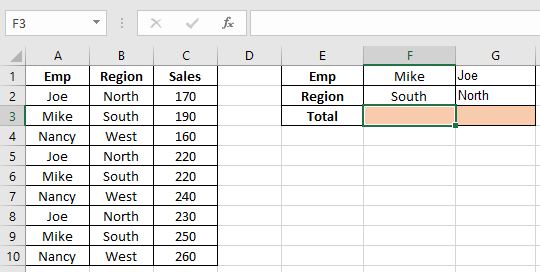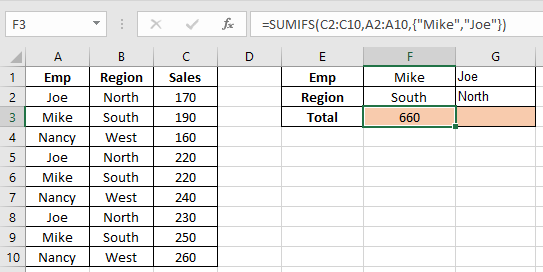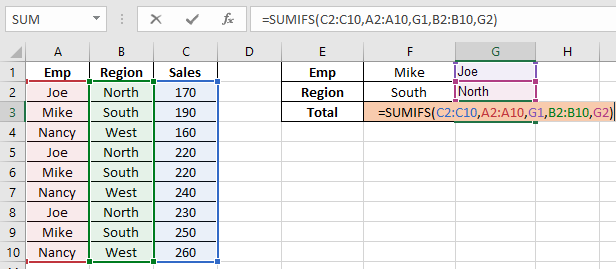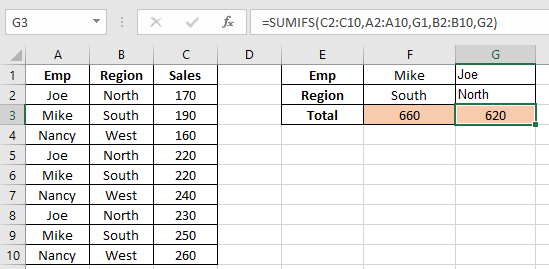# SUMIFS using AND-OR logic in Excel

In this article, we will learn how to use AND - OR logic in SUMIFS function in Excel.

AND logic is used when all conditions stated need to be satisfied.
OR logic is used when any condition stated satisfies.

In simple words, Excel lets you perform these both logic in SUMIFS function.

## SUMIFS with Or

OR logic with SUMIFS is used when we need to find the sum if value1 or value2 condition satisfy
Syntax of SUMIFS with OR logic

=SUM(SUMIFS ( sum_range, criteria_range, { "value1", "value2" }))Here we need to find the sum of Sales range If “Mike” or “Joe” occurs in Emp range
Use

=SUM(SUMIFS(C2:C10,A2:A10,{"Mike","Joe"}))As you can see we got the sum of combined Joe’s and Mike’s sales.

## SUMIFS with AND

AND logic with SUMIFS function is used when we need to find the sum if value1 and value2 both condition satisfy
Syntax of SUMIFS with AND logic

=SUMIFS ( sum_range, criteria_range1, value1, [criteria_range2, value2],.. )Here we need to find the sum of Sales range If “Joe” occurs in Emp range and “North” occurs in the Region range
Use

=SUMIFS ( C2:C10, A2:A10, G1, B2:B10, G2)As you can see we got the sum of just Joe’ North region.

Hope you understood how to use SUMIFS function with AND-OR logic in Excel. Explore more Mathematical functions with logic_test articles on Excel TEXT function here. Please feel free to state your query or feedback for the above article.

Related Articles:

3 SUMIF examples with Or Formula in Excel

How to Use SUMIF Function in Excel

How to Use SUMIFS Function in Excel

How to Sum if cells contain specific text in Excel

How to Sum If Greater Than 0 in Excel

SUMIFS with dates in Excel

Popular Articles:

50 Excel Shortcuts to Increase Your Productivity

How to use the VLOOKUP Function in Excel

How to use the COUNTIF function in Excel

How to use the SUMIF Function in Excel

Terms and Conditions of use

The applications/code on this site are distributed as is and without warranties or liability. In no event shall the owner of the copyrights, or the authors of the applications/code be liable for any loss of profit, any problems or any damage resulting from the use or evaluation of the applications/code.